# LU Decomposition Notes | Study GATE Computer Science Engineering(CSE) 2023 Mock Test Series - GATE

## GATE: LU Decomposition Notes | Study GATE Computer Science Engineering(CSE) 2023 Mock Test Series - GATE

The document LU Decomposition Notes | Study GATE Computer Science Engineering(CSE) 2023 Mock Test Series - GATE is a part of the GATE Course GATE Computer Science Engineering(CSE) 2023 Mock Test Series.
All you need of GATE at this link: GATE

L U Decomposition of a System of Linear Equations

L U decomposition of a matrix is the factorization of a given square matrix into two triangular matrices, one upper triangular matrix and one lower triangular matrix, such that the product of these two matrices gives the original matrix. It was introduced by Alan Turing in 1948, who also created the turing machine.

This method of factorizing a matrix as a product of two triangular matrices has various applications such as solution of a system of equations, which itself is an integral part of many applications such as finding current in a circuit and solution of discrete dynamical system problems; finding the inverse of a matrix and finding the determinant of the matrix.
Basically, the L U decomposition method comes handy whenever it is possible to model the problem to be solved into matrix form. Conversion to the matrix form and solving with triangular matrices makes it easy to do calculations in the process of finding the solution.

A square matrix A can be decomposed into two square matrices L and U such that A = L U where U is an upper triangular matrix formed as a result of applying Gauss Elimination Method on A; and L is a lower triangular matrix with diagonal elements being equal to 1.

For A =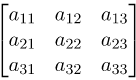, we have L =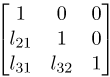and U =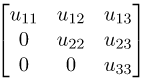; such that A = L U.

Gauss Elimination Method
According to the Gauss Elimination method:
1. Any zero row should be at the bottom of the matrix.
2. The first non zero entry of each row should be on the right hand side of the first non zero entry of the preceding row. This method reduces the matrix to row echelon form.

Steps for L U Decomposition
Given a set of linear equations, first convert them into matrix form A X = C where A is the coefficient matrix, X is the variable matrix and C is the matrix of numbers on the right hand side of the equations.

Now, reduce the coefficient matrix A , i.e., matrix obtained from the coefficients of variables in all the given equations such that for ‘n’ variables we have an nXn matrix, to row echelon form using Gauss Elimination Method. The matrix so obtained is U.

To find L, we have two methods. The first one is to assume the remaining elements as some artificial variables, make equations using A = L U and solve them to find those artificial variables.
The other method is that the remaining elements are the multiplier coefficients because of which the respective positions became zero in the U matrix. (This method is a little tricky to understand by words but would get clear in example below)

Now, we have A (the nXn coefficient matrix), L (the nXn lower triangular matrix), U (the nXn upper triangular matrix), X (the nX1 matrix of variables) and C (the nX1 matrix of numbers on the right hand side of the equations).

The given system of equations is A X = C. We substitute A = L U. Thus, we have L U X = C.
We put Z = U X, where Z is a matrix or artificial variables and solve for L Z = C first and then solve for U X = Z to find X or the values of the variables, which was required.

Example:
Solve the following system of equations using LU Decomposition method: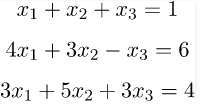Solution: Here, we have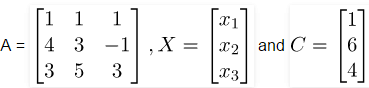such that A X = C.

Now, we first consider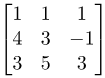and convert it to row echelon form using Gauss Elimination Method.

So, by doing   R2→R2 - 4R...................................................(1)
R3→R3 - 3R1....................................................(2)

we get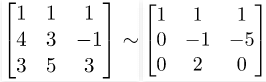R3→R- (- 2) R2.........................................................(3)

we get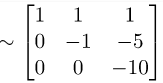(Remember to always keep ‘ – ‘ sign in between, replace ‘ + ‘ sign by two ‘ – ‘ signs)

Hence, we get L =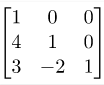and U =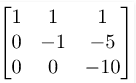(notice that in L matrix,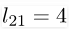is from (1),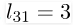is from (2) and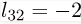is from (3))

Now, we assume Z =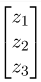and solve L Z = C.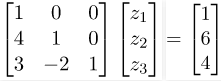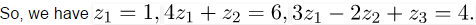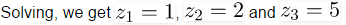Now, we solve U X = Z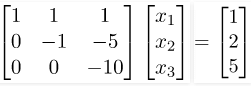Therefore, we get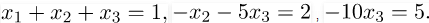Thus, the solution to the given system of linear equations is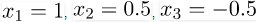and hence the matrix X =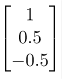The document LU Decomposition Notes | Study GATE Computer Science Engineering(CSE) 2023 Mock Test Series - GATE is a part of the GATE Course GATE Computer Science Engineering(CSE) 2023 Mock Test Series.
All you need of GATE at this link: GATEUse Code STAYHOME200 and get INR 200 additional OFF

## GATE Computer Science Engineering(CSE) 2023 Mock Test Series

136 docs|165 tests

Track your progress, build streaks, highlight & save important lessons and more!

,

,

,

,

,

,

,

,

,

,

,

,

,

,

,

,

,

,

,

,

,

;